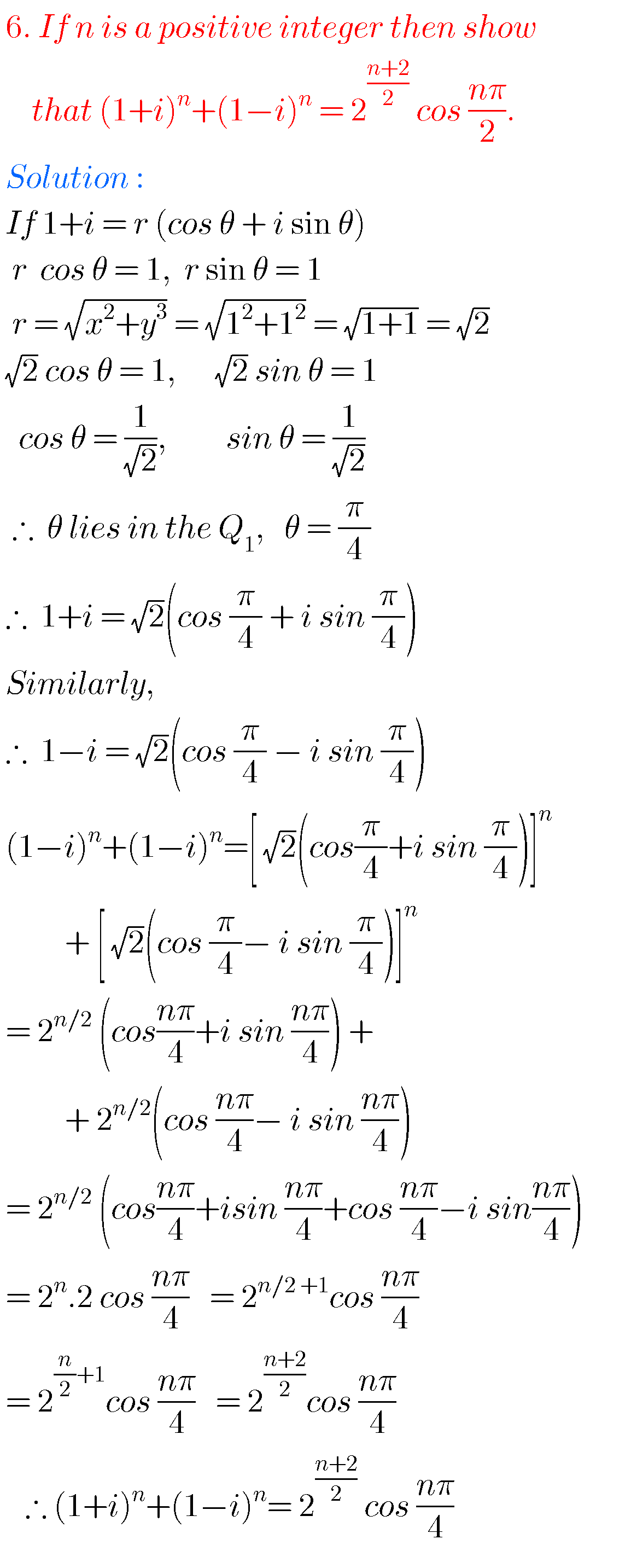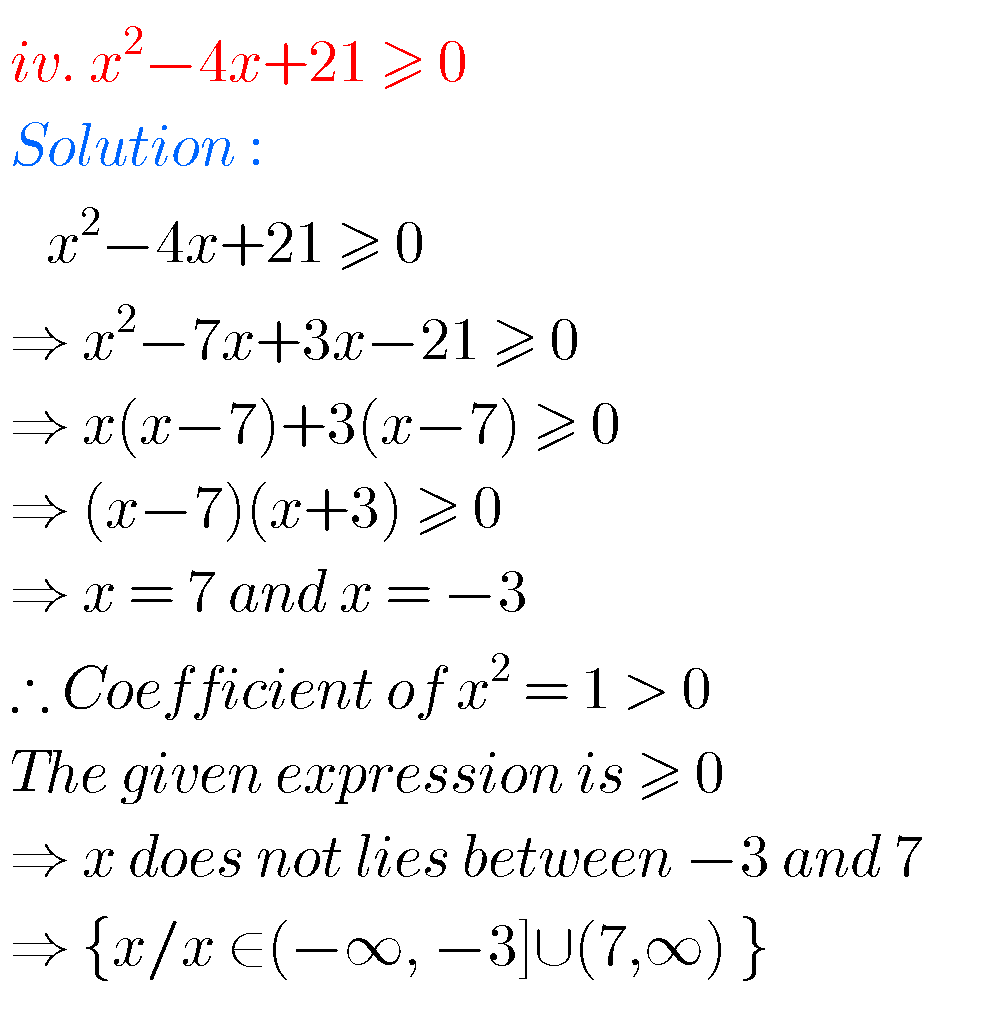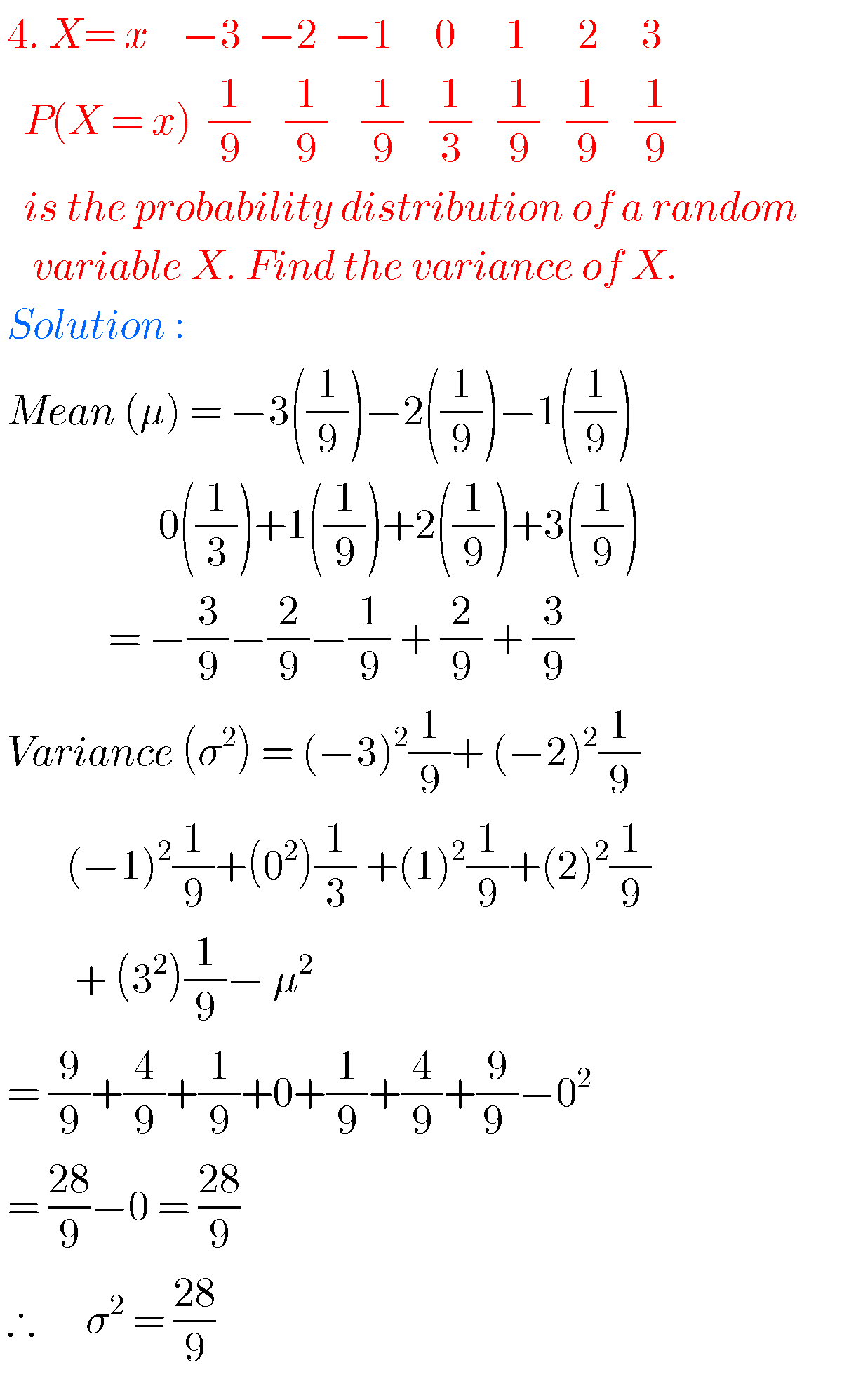# second inter maths solutions## Measures of dispersion solutions inter maths IIA,mathematics intermediate 2(a) chpater 8 exercise 8(a)

Measures of dispersion solutions inter maths IIA Intermediate mathematics IIA solutions for chapter 8, Measures of dispersion exercises 8(a) Read the text book very well. Then solve the example problems. The given solutions are very easy to understand. Observe the solutions and try them in your own method. You can also see the solutions for …## Binomial theorem solutions inter second year maths

Binomial theorem solutions inter second year maths Intermediate mathematics IIA chapter 6 Binomial theorem exercises 6(a), 6(b) and 6(c) solutions are given. These solutions are very easy to understand. First study the text book lessons very well. Then observe solutions and try them in your own method. You can also see the solutions for  inter …## Inter maths solutions for Quadratic expressions second year,intermediate maths 2a(IIA) chapter 3 exercise 3(a) text book solutions

Inter maths solutions for Quadratic expressions second year Intermediate mathematics second year solutions for quadratic expressions for some problems. These solutions are very easy to understand. These are helpful for examination purpose.  You can see the solutions for maths IIA inter  1. Complex numbers 2. De Mouvre’s theorem 3. Quadratic expressions exercise 3(a) Exercise 3(b) Exercise 3(c) 4. Theory …## Inter maths solution for De Moivre’s theorem,intermediate 2nd year mathematics IIA(2a) chapter 2 solutions

Inter maths solution for De Moivre’s theorem Mathematics intermediate second year maths IIA solutions for some problems. These are very easy to understand. Inter maths solutions for first year 1a and 1b for examination purpose. Inter maths 2a solutions for complex numbers and de moivre’s theorem. Inter first year 1a and 1b solutions are also …## Inter maths solution for complex numbers,intermediate 2nd year maths 2a chapter 1 solutions

Inter maths solutions for IIA complex numbers Intermediate 2nd year maths chapter 1 solutions for some problems. These solutions are very easy to understand. You can see the solutions for inter 1a 1. Functions 2. Mathematical induction 3. Matrices 4. Addition of vectors 5. Trigonometric ratios upto transformations 1 6. Trigonometric ratios upto transformations 2 7. Trigonometric equations 8. Inverse trigonometric functions 9. Hyperbolic …## Inter maths IIA solutions for quadratic expressions exercise 3(c),chapter 3 intermediate 2nd year 2a

Inter maths IIA solutions for quadratic expressions exercise 3(c) Intermediate second year mathematics quadratic expressions exercise 3(c) solutions ( for text book problems) are given below. These solutions are very easy to understand. You can also see the solurions for first year maths 1A and 1B for examination purpose. Inter maths 1A solutions   1. Functions 2. Mathematical …## Inter maths solutions for quadratic expressions,exercise 3(b) chapter 3 second year inter maths IIA

Inter maths solurions for quadratic expressions Intermediate second year mathematics IIA quadratic equations text book exercise 3(b) solutions are given. These solutions are very easy to understand. Study the text book. Observe the solutions and try them in your own methods  You can see the solutions for maths IIA inter  1. Complex numbers 2. De Mouvre’s theorem …## Inter maths solutions for IIA random variables and probability distributions

Inter maths solutions for IIA random variables and probability distributions Intermediate mathematics IIA chapter 10 random variables and probability distrbutions exercises 10(a) and 10(b) solutions are given. These solutions are very easy to understand. First study the text book lessons very well. Then observe solutions and try them in your own method. You can also …## Inter maths solutions for Theory of Equations,exercise 4(a),4(b),4(c),4(d) chapter 4 intermediate maths IIA

Inter maths solutions for Theory of Equations Intermediate mathematics IIA chapter 4 theory of equations exercises 4(a), 4(b), 4(c) and 4(d) (text book) solutions are given. These solutions are very easy to understand. First study the text book lessons very well. Then observe solutions and try them in your own method. You can also see …## Inter maths solutions for Permutations and Combinations,exercise 5(a),5(b),5(c),5(d),5(e) chapter 5 intermediate

Inter maths solutions for Permutations and Combinations Text book exercises solutions for Permutations and Combinations second inter maths IIA. These solutions are very easy to understand. Exercises 5(a), 5(b), 5(c), 5(d) and 5(e) solutions are given. You can also see the solutions for 1. Complex numbers 2. De Moivre’s theorem 3. Quadratic equations Exercise 3(a) Exercise 3(b) Exercise …